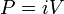# Abvolt

The abvolt (symbol abV) is the unit of electric potential difference (electromotive force) in the cgs-emu (centimeter-gram-second electromagnetic) system of units.

The abvolt is defined as the difference in electric potential between two points in a conductor carrying a constant current of 1 abampere ( = 10 A) when the power dissipated between them is equal to 1 erg ( = 10−7 joule) per second. From$\, P = i V$

with power P in erg/s and electric current i in abA, we obtain by dimensional analysis

1 abvolt = 1 erg/(s⋅abA) = 10−7 J/(10⋅s⋅A) = 1⋅10−8 J/C = 1⋅10−8 volt.